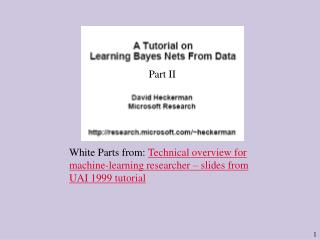DownloadDownload PresentationPart II

# Part II

Download Presentation## Part II

- - - - - - - - - - - - - - - - - - - - - - - - - - - E N D - - - - - - - - - - - - - - - - - - - - - - - - - - -
##### Presentation Transcript

1. Part II White Parts from: Technical overview for machine-learning researcher – slides from UAI 1999 tutorial

2. = Ct,h Example: for (ht + htthh), we get p(d|m) = 3!2!/6!

3. Numerical example for the network X1 X2 Imaginary sample sizes denoted N’ijk Data: (true, true) and (true, false)

4. Used so far Desired

5. How do we assign structure and parameter priors ? Structure priors: Uniform, partial order (allowed/prohibited edges), proportional to similarityto some a priori network.

6. BDe K2

7. So how to generate parameter priors? Example: Suppose the hyper distribution for (X1,X2) is Dir( a00, a01 ,a10, a11).

8. Example: Suppose the hyper distribution for (X1,X2) is Dir( a00, a01 ,a10, a11) This determines a Dirichlet distribution for the parameters of both directed models.

9. Summary: Suppose the parameters for (X1,X2) are distributed Dir( a00, a01 ,a10, a11). Then, parameters for X1 are distributed Dir(a00+a01 ,a10+a11). Similarly, parameters for X2 are distributed Dir(a00+a10 ,a01+a11).

10. BDe score:

11. Functional Equations Example • Example: f(x+y) = f(x) f(y) • Solution: (ln f )`(x+y) = (ln f )`(x) • and so: (ln f )`(x) = constant • Hence: (ln f )(x) = linear function • hence: f(x) = c eax • Assumptions: Positive everywhere, Differentiable

12. The bivariate discrete case

13. The bivariate discrete case

14. The bivariate discrete case

15. The bivariate discrete case